Open In App
Related Articles
• CBSE Class 9 Maths Revision Notes
• CBSE Class 9 Maths Formulas
• NCERT Solutions for Class 9 Maths
• RD Sharma Class 9 Solutions

# Types of Angles

Angles are an essential and fundamental concept in the field of geometry which is the branch of mathematics that deals with the relationship of points, lines, angles, and shapes in space. Understanding different types of angles is crucial for either solving theoretical problems in geometry or any DIY project you are doing to satisfy your curiosity. As we know that an angle is a geometrical shape formed by joining two rays at their endpoints and is generally measured in degrees. There exist different types of angles in geometry which we will discuss further in this article.

Other than that, In this article, we will explore the various types of angles and provide clear explanations, diagrams, and examples to help you master this fundamental concept.

## What are Angles?

Angles are a mathematical concept used to measure the number of turns between two lines or line segments that meet at a common point. They are typically measured in degrees or radians, and are often represented by a symbol like the letter “θ”. In Euclidean geometry, an angle is defined as a figure that is formed by two rays (by their intersection).

The rays are known as the sides of the angle and the common point shared by the two rays is known as the vertex of the angle. Angle tells about the rotation and the amount of rotation of a certain parameter. Therefore, in physics, angular displacement and the terms used in the rotation part are concerned with angles.

### Parts of an Angle

The two main parts related to an angle are – the arms and the vertex.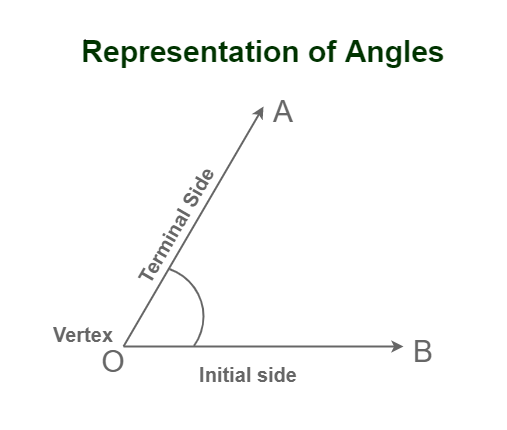Arms of the Angle: The two rays which join at a common point to form the angle are called the arms of the angle. Observe the figure above which shows that OA and OB are the arms of the angle AOB.

Vertex of the Angle: Vertex is a common endpoint that is shared by the two rays. Observe the figure in which the vertex O is marked as the joining point of the two arms.

## Types of Angles Based on Measurement

Measurement of angles is useful to differentiate types of angles. On the basis of their measurement, there are six different types of Angles commonly used in Mathematics:

• Acute Angle
• Right Angle
• Obtuse Angle
• Straight Angle
• Reflex Angle
• Complete Angle

Below is an explanation of each of the different types of angles with their figures:

### Acute Angle

When the two rays make any inclination between zero to ninety degrees it is called an Acute angle i.e. 0° < Angle < 90°. The corners of an acute angle triangle, the tip of a pencil, or the point of a star are some examples of acute angles. Here, the angle given below is an acute angle.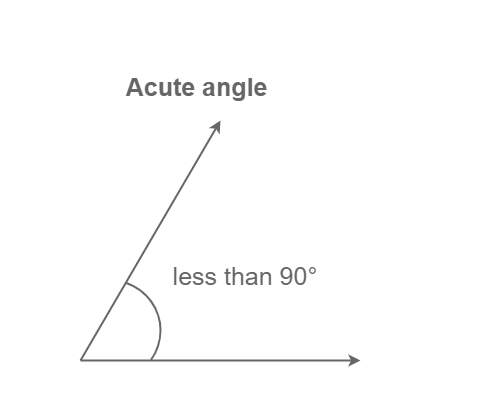### Right Angle

When the two rays make any inclination of exactly nighty degrees it is called a Right angle i.e. Angle = 90°. You can find it in many everyday objects, like the corners of a book or the edge of a door frame. Here, the angle given below is a right angle.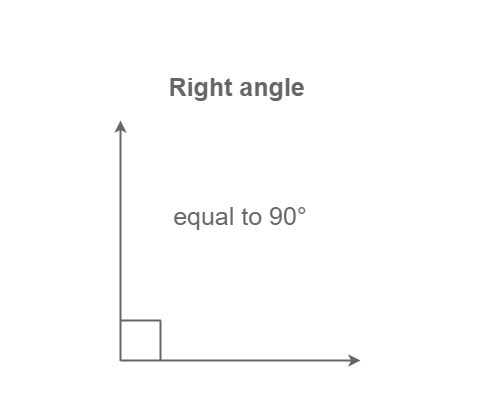### Obtuse Angle

When the two rays make any inclination of exactly nighty degrees it is called an Obtuse angle i.e. 90° < Angle < 180°. The largest angle of the obtuse angle tangle is a key example of the obtuse angles. Here, the angle given below is a right angle.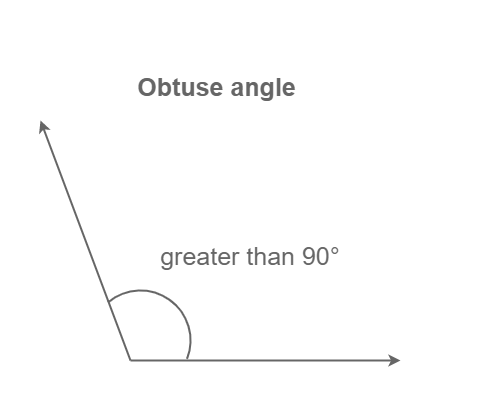### Straight Angle

When the two rays make any inclination of exactly 180 degrees it is called a Straight angle i.e. Angle = 180°. A straight angle is used to straight edge which is used to align something parallel to the horizontal. Here, the angle given below is a straight angle.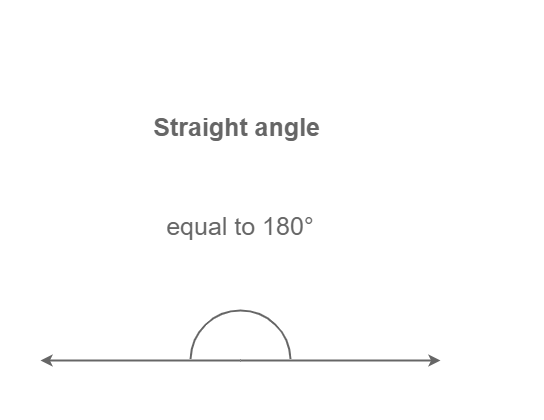### Reflex Angle

When the two rays make any inclination of greater than 180 degrees but less than 270 degrees. It is called a reflexive angle i.e. 180° < Angle < 270°. The exterior angle of any basic regular polygon is a reflex angle. Here, the angle given below is a reflexive angle.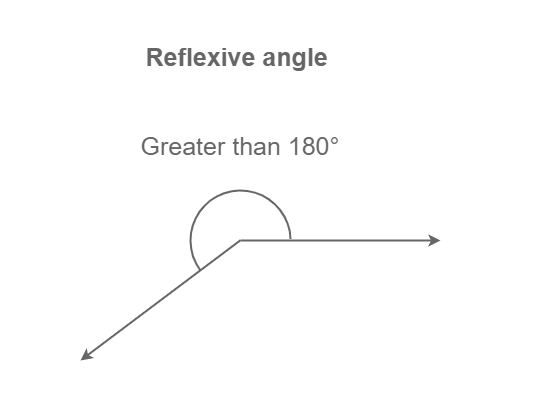### Complete Angle

When the two rays make any inclination of 360° i.e. they overlap each other. It is called a complete angle i.e. Angle = 360°. Here, the angle given below is a complete angle.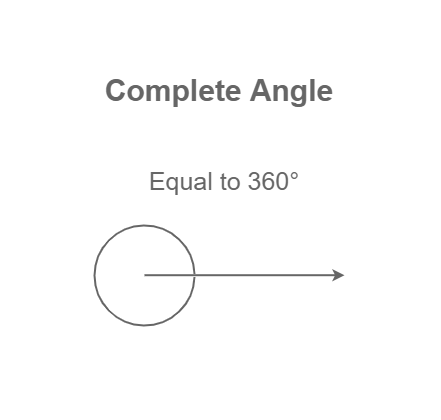## Types of Angles based on Rotation

On the basis of measurement of angles or rotation of angles can be two types:

• Positive Angles
• Negative Angles

### Positive Angles

Angles that are measured in a counterclockwise direction from the base are called Positive angles. Generally, positive angles are used to represent angles in geometry. From the origin, if an angle is drawn in the First Quadrant, it forms a positive angle.

### Negative Angles

Angles that are measured in a clockwise direction from the base are called Negative angles. From the origin, if an angle is drawn in the Third Quadrant, it forms a negative angle.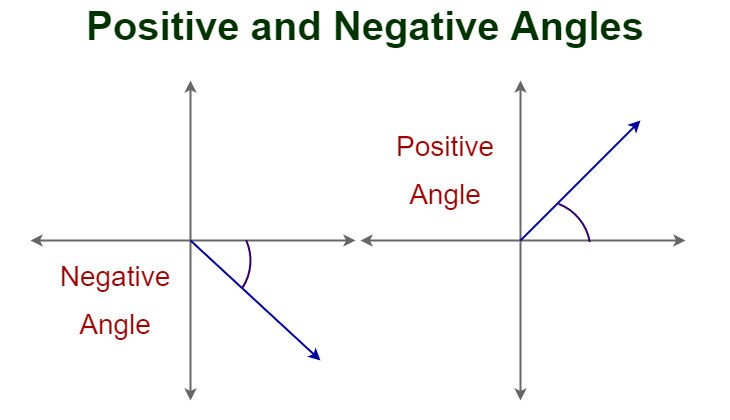## Types of Pair of Angles

Based on the sum of pairs, two angles pairs can be classified as

• Linear Pair
• Vertically Opposite Angles
• Complementary Angles
• Supplementary Angles

Two angles are called adjacent angles if they share only one common arm. In other words, adjacent angles are those two angles that have a common vertex and a common side but no common interior points. The sum of adjacent angles is equal to the measure of the larger angle formed by the two adjacent angles.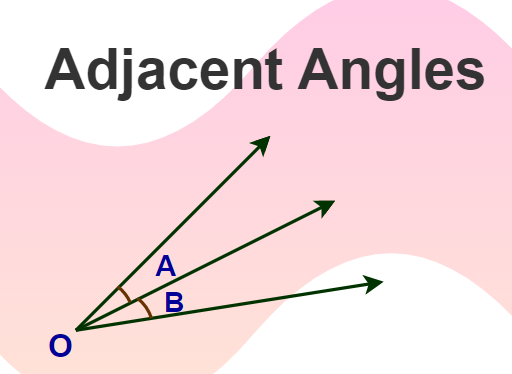### Linear Pair

A linear pair refers to a pair of adjacent angles whose non-common sides are opposite rays. In other words, two angles are said to form a linear pair if their sum is equal to 180°. For example, angles 70° and 110° form a linear pair because they are adjacent angles (share a common vertex and side) and their non-common sides form a straight line.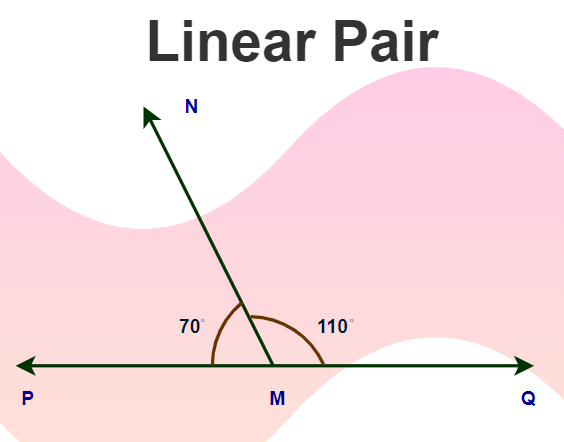### Vertically Opposite Angles

Vertically opposite angles are a pair of angles formed when two lines intersect. These angles have the same measure, and their opposite rays form a straight line. Vertically opposite angles are always congruent, and the measure of one angle can be used to find the measure of the other angle.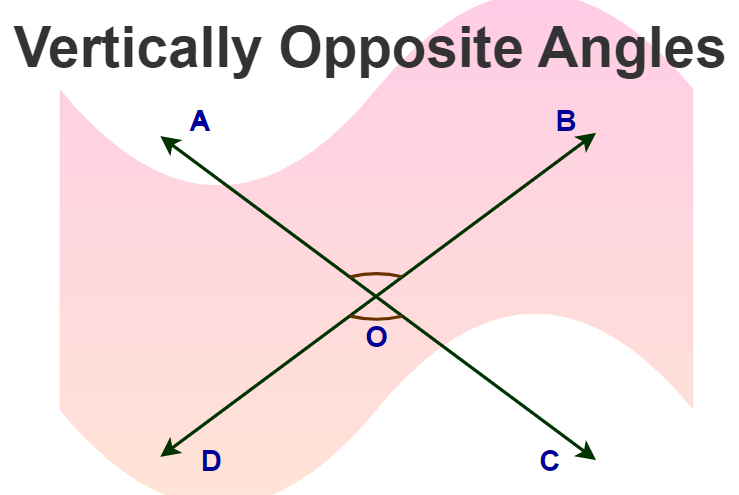### Complementary Angles

If the sum of two angles is equal to 90°, they are termed complementary angles. Two angles are complementary if they sum up to 90°. For example, 40° and 50° are complementary angles as 40° + 50° = 90°. Here, one angle complements the other angle.

### Supplementary Angles

If the sum of two angles is equal to 180°, they are termed supplementary angles. Two angles are supplementary if they sum up to 90°. For example, 140° and 40° are supplementary angles as 140° + 40° = 180°. Here, one angle supplements the other angle.

## Applications of Angles

As angles are purely mathematical concepts but they can be used for many real-life applications such as geometry, trigonometry, navigation, cartography, architecture, and engineering. Let’s understand these applications are as follows:

• Geometry and Trigonometry: Geometry and trigonometry may not be everyone’s favourite subjects, but they have a lot of real-world applications. Angles are used to measure the size and shape of objects, like circles, triangles, and polygons. Trigonometric functions, like sine, cosine, and tangent, are used to calculate distances using angles in fields like physics and engineering.
• Navigation and Cartography: Angles are critical in navigation and cartography as well as they help us determine direction, elevation, and position relative to other objects.
• Architecture and Engineering: Angles are essential tools in the field of architecture and engineering as different types of angles help us measure the slope of roofs, the angle of buildings, and the pitch of stairs. In engineering, angles are used to calculate various things such as the strength of materials, like steel beams, that are used in construction projects and also help engineers to design equipment, like gears and pulleys.

## Solved Examples on Types of Angles

Example 1: An Angle A measures 135° in measure. Which type of category does Angle A fall into?

Angle A lies between 90° to 180° in measure. Angle A is, therefore, an Obtuse Angle.

Example 2: An Angle S measures 35° in measure. Which type of category does Angle S fall into?

Angle S lies between 0° to 90° in measure. Angle S is therefore an Acute Angle.

Example 3: An Angle X measures 235 degrees in measure. Which type of category does Angle X fall into?

Angle X lies between 180° to 360° in measure. Angle X is therefore a Reflex Angle.

Example 4: An Angle Z measures 335° in measure. Which type of category does Angle Z fall into?

Angle Z lies between 180° to 360° in measure. Angle Z is therefore a Reflex Angle.

Example 5: Find the complement of 40° and the supplement of 25°.

Solution:

Those  angles that sum up to 90°are called as Complementary angles .

The complement of 40° is 50° because (90° – 40° = 50°).

Those  angles that sum up to 180°are called as Supplementary angles.

The supplement of 25° is 155° because (180° – 25° = 155°).

## FAQs on Types of Angles

### Q1: What is an Angle?

Figure formed by two rays and a point in geometry is called an angle, where rays are called arms of angle and the point is called vertex of angle.

### Q2: What are the Types of Angles Based on Rotation?

On the basis of rotation, types of angles are classified into:

• Positive Angles
• Negative Angles

### Q3: What are the Six Different Types of Angles Used in Geometry?

The six different angles used in geometry are:

• Acute Angle
• Obtuse Angle
• Right Angle
• Straight Angle
• Reflex Angle
• Complete Angle

### Q4: What are the Types of Triangles on the Basis of Angles?

On the basis of angles, Triangles are classified as:

• Acute Angled Triangle
• Obtuse Angled Triangle
• Right Angled Triangle

### Q5: What is an Acute Angle?

An acute angle is an angle that measures between 0 and 90 degrees.

### Q6: What is a Right Angle?

A right angle is an angle that measures exactly 90 degrees.

### Q7: What is an Obtuse Angle?

An obtuse angle is an angle that measures between 90 and 180 degrees.

### Q8: What is a Reflex Angle?

A reflex angle is an angle that measures between 180 and 360 degrees.